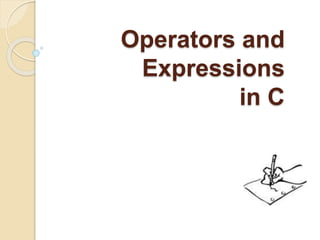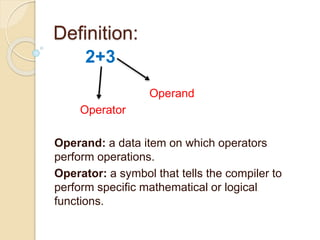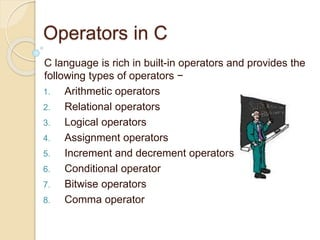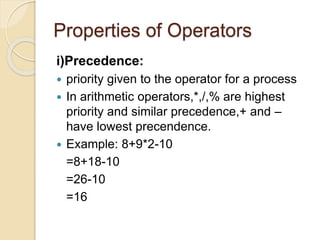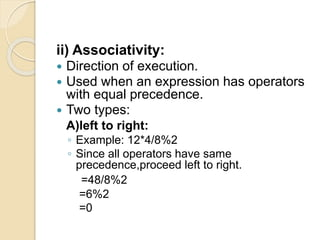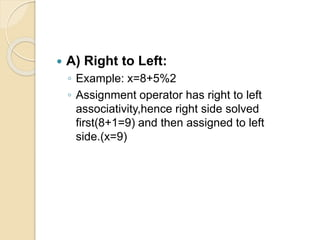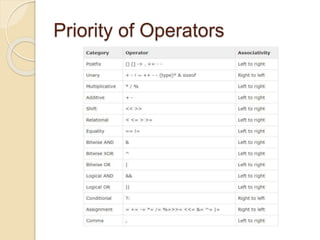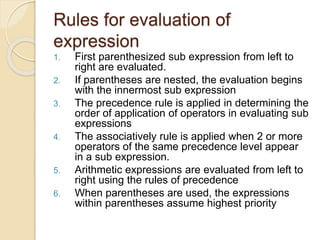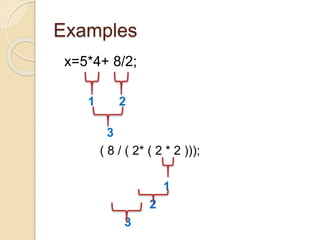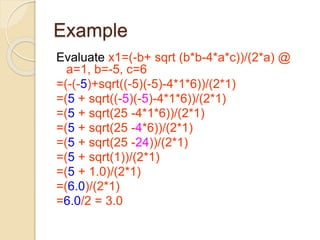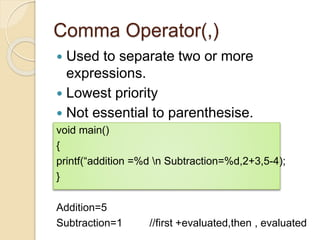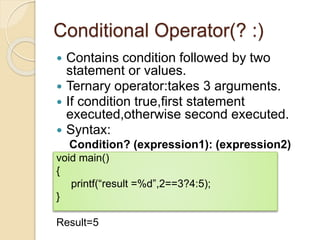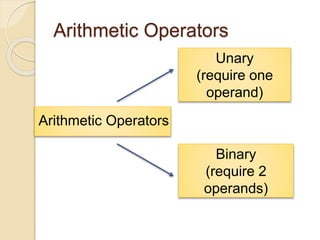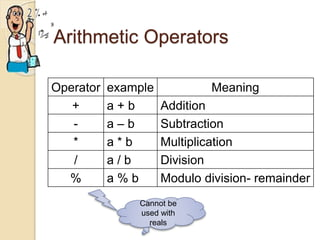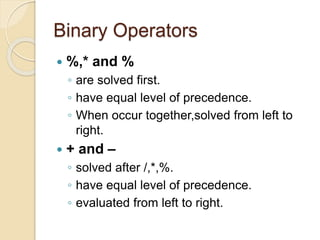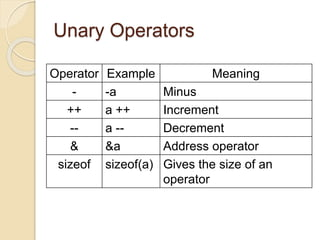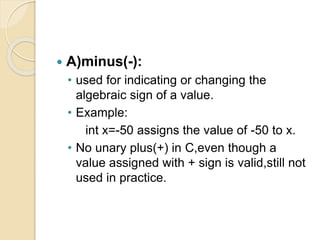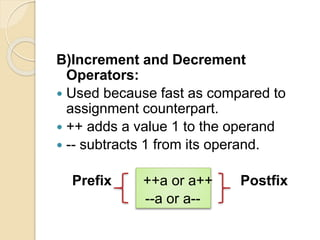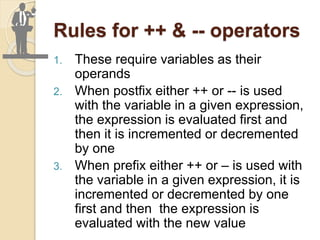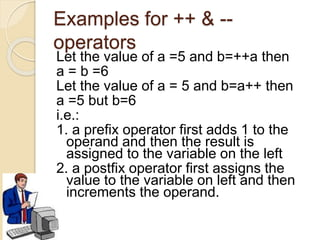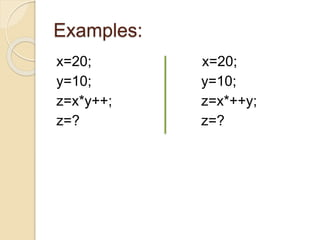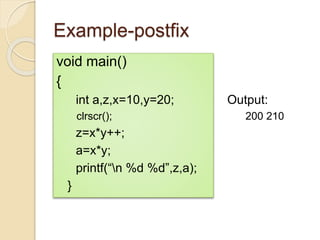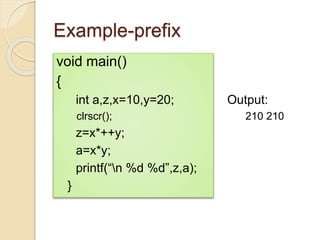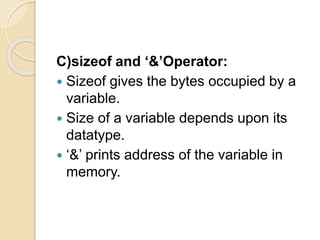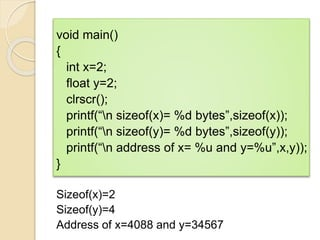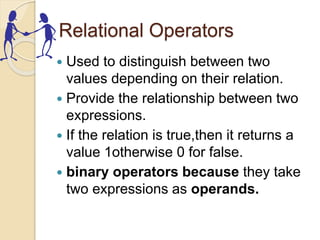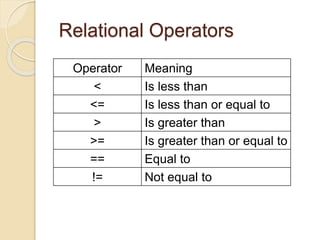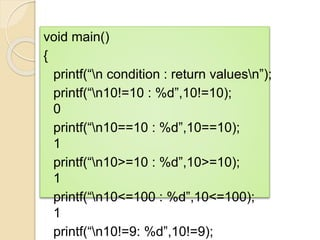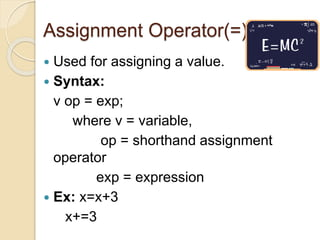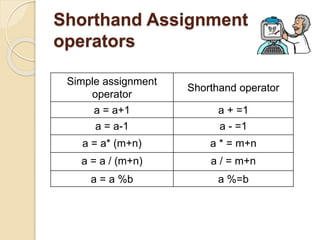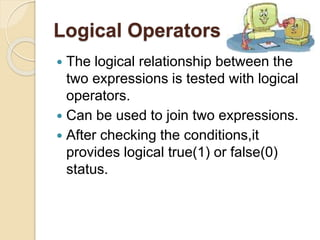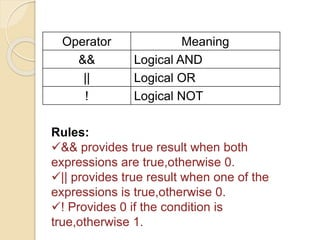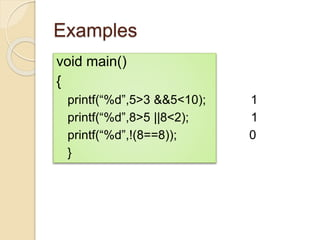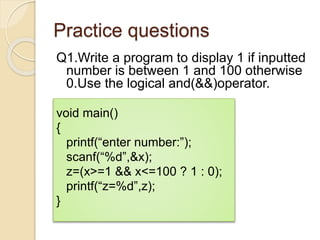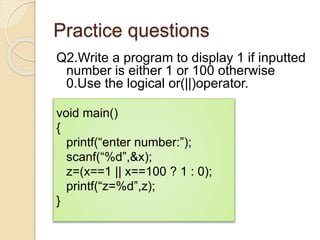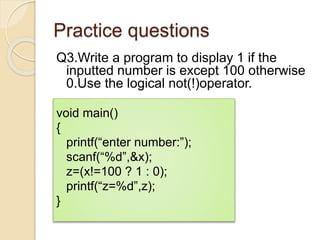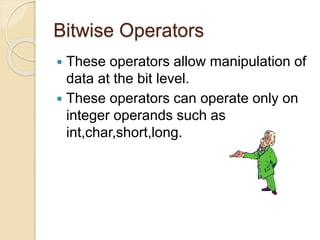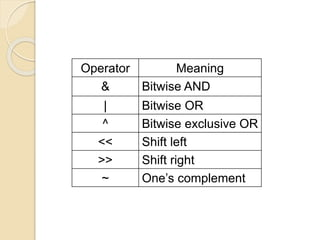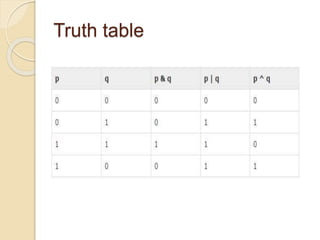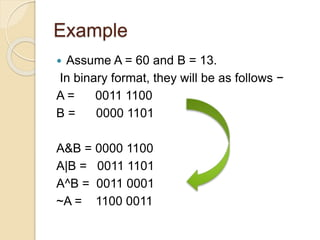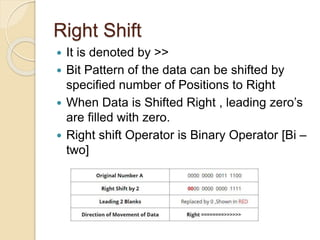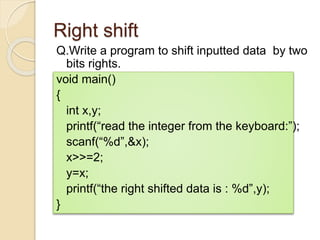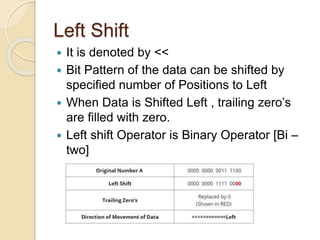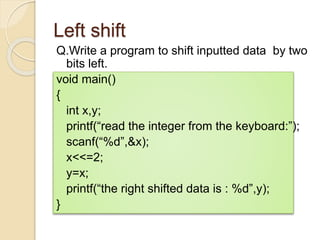1 of 46

### Operators and expressions in c language

1. Operators and Expressions in C
2. 2+3 Operand Operator Operand: a data item on which operators perform operations. Operator: a symbol that tells the compiler to perform specific mathematical or logical functions. Definition:
3. Operators in C C language is rich in built-in operators and provides the following types of operators − 1. Arithmetic operators 2. Relational operators 3. Logical operators 4. Assignment operators 5. Increment and decrement operators 6. Conditional operator 7. Bitwise operators 8. Comma operator
4. Properties of Operators i)Precedence:  priority given to the operator for a process  In arithmetic operators,*,/,% are highest priority and similar precedence,+ and – have lowest precendence.  Example: 8+9*2-10 =8+18-10 =26-10 =16
5. ii) Associativity:  Direction of execution.  Used when an expression has operators with equal precedence.  Two types: A)left to right: ◦ Example: 12*4/8%2 ◦ Since all operators have same precedence,proceed left to right. =48/8%2 =6%2 =0
6.  A) Right to Left: ◦ Example: x=8+5%2 ◦ Assignment operator has right to left associativity,hence right side solved first(8+1=9) and then assigned to left side.(x=9)
7. Priority of Operators
8. Rules for evaluation of expression 1. First parenthesized sub expression from left to right are evaluated. 2. If parentheses are nested, the evaluation begins with the innermost sub expression 3. The precedence rule is applied in determining the order of application of operators in evaluating sub expressions 4. The associatively rule is applied when 2 or more operators of the same precedence level appear in a sub expression. 5. Arithmetic expressions are evaluated from left to right using the rules of precedence 6. When parentheses are used, the expressions within parentheses assume highest priority
9. Examples x=5*4+ 8/2; 1 2 3 ( 8 / ( 2* ( 2 * 2 ))); 1 2 3
10. Example Evaluate x1=(-b+ sqrt (b*b-4*a*c))/(2*a) @ a=1, b=-5, c=6 =(-(-5)+sqrt((-5)(-5)-4*1*6))/(2*1) =(5 + sqrt((-5)(-5)-4*1*6))/(2*1) =(5 + sqrt(25 -4*1*6))/(2*1) =(5 + sqrt(25 -4*6))/(2*1) =(5 + sqrt(25 -24))/(2*1) =(5 + sqrt(1))/(2*1) =(5 + 1.0)/(2*1) =(6.0)/(2*1) =6.0/2 = 3.0
11. Comma Operator(,)  Used to separate two or more expressions.  Lowest priority  Not essential to parenthesise. void main() { printf(“addition =%d n Subtraction=%d,2+3,5-4); } Addition=5 Subtraction=1 //first +evaluated,then , evaluated
12. Conditional Operator(? :)  Contains condition followed by two statement or values.  Ternary operator:takes 3 arguments.  If condition true,first statement executed,otherwise second executed.  Syntax: Condition? (expression1): (expression2) void main() { printf(“result =%d”,2==3?4:5); } Result=5
13. Arithmetic Operators Arithmetic Operators Unary (require one operand) Binary (require 2 operands)
14. Arithmetic Operators Operator example Meaning + a + b Addition - a – b Subtraction * a * b Multiplication / a / b Division % a % b Modulo division- remainder Cannot be used with reals
15. Binary Operators  %,* and % ◦ are solved first. ◦ have equal level of precedence. ◦ When occur together,solved from left to right.  + and – ◦ solved after /,*,%. ◦ have equal level of precedence. ◦ evaluated from left to right.
16. Unary Operators Operator Example Meaning - -a Minus ++ a ++ Increment -- a -- Decrement & &a Address operator sizeof sizeof(a) Gives the size of an operator
17.  A)minus(-): • used for indicating or changing the algebraic sign of a value. • Example: int x=-50 assigns the value of -50 to x. • No unary plus(+) in C,even though a value assigned with + sign is valid,still not used in practice.
18. B)Increment and Decrement Operators:  Used because fast as compared to assignment counterpart.  ++ adds a value 1 to the operand  -- subtracts 1 from its operand. Prefix ++a or a++ Postfix --a or a--
19. Rules for ++ & -- operators 1. These require variables as their operands 2. When postfix either ++ or -- is used with the variable in a given expression, the expression is evaluated first and then it is incremented or decremented by one 3. When prefix either ++ or – is used with the variable in a given expression, it is incremented or decremented by one first and then the expression is evaluated with the new value
20. Examples for ++ & -- operators Let the value of a =5 and b=++a then a = b =6 Let the value of a = 5 and b=a++ then a =5 but b=6 i.e.: 1. a prefix operator first adds 1 to the operand and then the result is assigned to the variable on the left 2. a postfix operator first assigns the value to the variable on left and then increments the operand.
21. Examples: x=20; x=20; y=10; y=10; z=x*y++; z=x*++y; z=? z=?
22. Example-postfix void main() { int a,z,x=10,y=20; Output: clrscr(); 200 210 z=x*y++; a=x*y; printf(“n %d %d”,z,a); }
23. Example-prefix void main() { int a,z,x=10,y=20; Output: clrscr(); 210 210 z=x*++y; a=x*y; printf(“n %d %d”,z,a); }
24. C)sizeof and ‘&’Operator:  Sizeof gives the bytes occupied by a variable.  Size of a variable depends upon its datatype.  ‘&’ prints address of the variable in memory.
25. void main() { int x=2; float y=2; clrscr(); printf(“n sizeof(x)= %d bytes”,sizeof(x)); printf(“n sizeof(y)= %d bytes”,sizeof(y)); printf(“n address of x= %u and y=%u”,x,y)); } Sizeof(x)=2 Sizeof(y)=4 Address of x=4088 and y=34567
26. Relational Operators  Used to distinguish between two values depending on their relation.  Provide the relationship between two expressions.  If the relation is true,then it returns a value 1otherwise 0 for false.  binary operators because they take two expressions as operands.
27. Relational Operators Operator Meaning < Is less than <= Is less than or equal to > Is greater than >= Is greater than or equal to == Equal to != Not equal to
28. void main() { printf(“n condition : return valuesn”); printf(“n10!=10 : %d”,10!=10); 0 printf(“n10==10 : %d”,10==10); 1 printf(“n10>=10 : %d”,10>=10); 1 printf(“n10<=100 : %d”,10<=100); 1 printf(“n10!=9: %d”,10!=9);
29. Assignment Operator(=)  Used for assigning a value.  Syntax: v op = exp; where v = variable, op = shorthand assignment operator exp = expression  Ex: x=x+3 x+=3
30. Shorthand Assignment operators Simple assignment operator Shorthand operator a = a+1 a + =1 a = a-1 a - =1 a = a* (m+n) a * = m+n a = a / (m+n) a / = m+n a = a %b a %=b
31. Logical Operators  The logical relationship between the two expressions is tested with logical operators.  Can be used to join two expressions.  After checking the conditions,it provides logical true(1) or false(0) status.
32. Operator Meaning && Logical AND || Logical OR ! Logical NOT Rules: && provides true result when both expressions are true,otherwise 0. || provides true result when one of the expressions is true,otherwise 0. ! Provides 0 if the condition is true,otherwise 1.
33. Truth Table a b Value of the expression a && b a || b 0 0 0 0 0 1 0 1 1 0 0 1 1 1 1 1
34. Examples void main() { printf(“%d”,5>3 &&5<10); 1 printf(“%d”,8>5 ||8<2); 1 printf(“%d”,!(8==8)); 0 }
35. Practice questions Q1.Write a program to display 1 if inputted number is between 1 and 100 otherwise 0.Use the logical and(&&)operator. void main() { printf(“enter number:”); scanf(“%d”,&x); z=(x>=1 && x<=100 ? 1 : 0); printf(“z=%d”,z); }
36. Practice questions Q2.Write a program to display 1 if inputted number is either 1 or 100 otherwise 0.Use the logical or(||)operator. void main() { printf(“enter number:”); scanf(“%d”,&x); z=(x==1 || x==100 ? 1 : 0); printf(“z=%d”,z); }
37. Practice questions Q3.Write a program to display 1 if the inputted number is except 100 otherwise 0.Use the logical not(!)operator. void main() { printf(“enter number:”); scanf(“%d”,&x); z=(x!=100 ? 1 : 0); printf(“z=%d”,z); }
38. Bitwise Operators  These operators allow manipulation of data at the bit level.  These operators can operate only on integer operands such as int,char,short,long.
39. Operator Meaning & Bitwise AND | Bitwise OR ^ Bitwise exclusive OR << Shift left >> Shift right ~ One’s complement
40. Truth table
41. Example  Assume A = 60 and B = 13. In binary format, they will be as follows − A = 0011 1100 B = 0000 1101 A&B = 0000 1100 A|B = 0011 1101 A^B = 0011 0001 ~A = 1100 0011
42. Right Shift  It is denoted by >>  Bit Pattern of the data can be shifted by specified number of Positions to Right  When Data is Shifted Right , leading zero’s are filled with zero.  Right shift Operator is Binary Operator [Bi – two]
43. Right shift Q.Write a program to shift inputted data by two bits rights. void main() { int x,y; printf(“read the integer from the keyboard:”); scanf(“%d”,&x); x>>=2; y=x; printf(“the right shifted data is : %d”,y); }
44. Left Shift  It is denoted by <<  Bit Pattern of the data can be shifted by specified number of Positions to Left  When Data is Shifted Left , trailing zero’s are filled with zero.  Left shift Operator is Binary Operator [Bi – two]
45. Left shift Q.Write a program to shift inputted data by two bits left. void main() { int x,y; printf(“read the integer from the keyboard:”); scanf(“%d”,&x); x<<=2; y=x; printf(“the right shifted data is : %d”,y); }